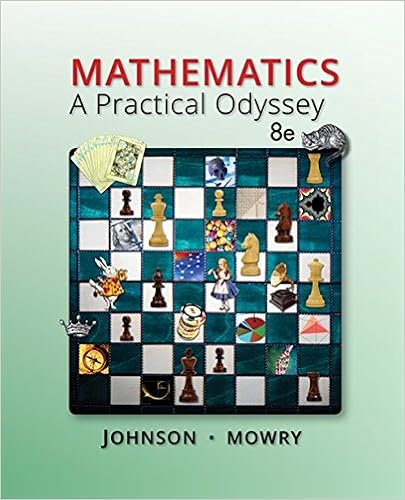# 15 if current prices break through the 50 day ma line

• Test Prep
• 367
• 88% (17) 15 out of 17 people found this document helpful

This preview shows page 167 - 171 out of 367 pages.

##### We have textbook solutions for you!
The document you are viewing contains questions related to this textbook.The document you are viewing contains questions related to this textbook.
Chapter 11 / Exercise 5
Mathematics: A Practical Odyssey
Johnson/MowryExpert Verified
15. If current prices break through the 50-day MA line from above on heavy volume, technicians would expect a reversal of a rising trend and become bearish. If the 50-day MA line crosses the 200-day MA line from above on good volume, technicians would again expect a reversal in rising prices and become bearish. 16. Relative strength is the ratio of a firm’s stock price to a market price series. If the relative strength ratio is increasing during a bear market it means that the stock is not declining as much as the aggregate market. Under such favorable conditions, the technician would expect the stock to likewise outperform the market in the ensuing bull market. 17. Technicians recognize that there is no single technical trading rule that is correct all the time - even the best ones miss certain turns or give false signals. Also, various indicators provide different information for alternative segments of the market. Therefore, you don't want to depend on any one technique, but look at several and derive a consensus.
##### We have textbook solutions for you!
The document you are viewing contains questions related to this textbook.The document you are viewing contains questions related to this textbook.
Chapter 11 / Exercise 5
Mathematics: A Practical Odyssey
Johnson/MowryExpert Verified
16 - 4 CHAPTER 16Answers to Problems1. Student Exercise 2. Student Exercise 3. Student Exercise 4. 40 39 38 X 0 37 X 0 36 X 0 35 X 0 34 X 0 33 X 0 32 X 0 31 X 0 30 X 0 X 29 X 0 X 28 X 0 X 27 X 0 X 26 X 0 X 25 X 24 X 23 X The price of the stock went to 38 1/2 before declining. Therefore, at the current price of around 30, the chart could imply a buying opportunity since the price has risen recently from 26 to 30 without a reversal. 5.a. Day 4 = (12,010 + 12,100 + 12,165 + 12,080)/4 = 48,355/4 = 12,088.75 Day 5 = (12,100 + 12,165 + 12,080 + 12,070)/4 = 48,415/4 = 12,103.75 Day 6 = (12,165 + 12,080 + 12,070 + 12,150)/4 = 48,465/4 = 12,116.25 Day 7 = (12,080 + 12,070 + 12,150 + 12,220)/4 = 48,520/4 = 12,130.00 Day 8 = (12,070 + 12,150 + 12,220 + 12,130)/4 = 48,570/4 = 12,142.50 Day 9 = (12,150 + 12,220 + 12,130 + 12,250)/4 = 48,750/4 = 12,187.50
16 - 5 Day 10 = (12,220 + 12,130 + 12,250 + 12,315)/4 = 48,915/4 = 12,228.75 Day 11 = (12,130 + 12,250 + 12,315 + 12,240)/4 = 48,935/4 = 12,233.75 Day 12 = (12,250 + 12,315 + 12,240 + 12,310)/4 = 49,115/4 = 12,278.75 5.b. This would indicate a the continuation of a bullish trend, because the index closed above its 4-day moving average for day 13 of 12,291.25, which it has done for the last several days. 6. Day 1 2 3 4 5Net advances +448 +95 +519 -499 -193 (advances minus declines) Cumulative net +448 +543 +1062 +563 +370 advances
17 - 1 CHAPTER 17 EQUITY PORTFOLIO MANAGEMENT STRATEGIES Answers to Questions1. Passive portfolio management strategies have grown in popularity because investors are recognizing that the stock market is fairly efficient and that the costs of an actively managed portfolio are substantial. 2. Numerous studies have shown that the majority of portfolio managers have been unable to match the risk-return performance of stock or bond indexes. Following an indexing portfolio strategy, the portfolio manager builds a portfolio that matches the performance of an index, thereby reducing the costs of research and trading. In an indexing strategy, the portfolio manager’s evaluation is based upon how closely the portfolio tracks the index or “tracking error,” rather than a risk-return performance evaluation. 3. There are a number of active management strategies discussed in the chapter including sector rotation, the use of factor models, quantitative screens, and linear programming methods.
•••